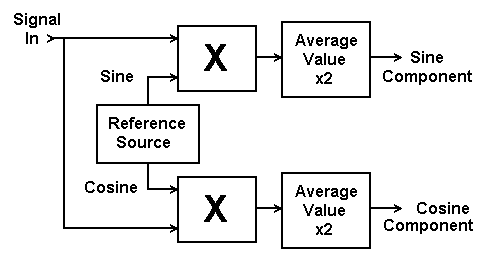Daqarta
Data AcQuisition And Real-Time Analysis
Scope - Spectrum - Spectrogram - Signal Generator
Software for WindowsThe following is from the Daqarta Help system:

# Practical Spectrum Analyzer

As we have seen, our Conceptual Spectrum Analyzer has serious problems if the input signal is out of phase with the reference signal, even if the frequencies are the same.

But all is not lost. While we have relied upon the formula for the product of two sine waves, it turns out there is a very similar formula for the product of two cosine waves, which differs only in that the cos(A+B) term of the product is positive instead of negative. We can thus analyze the unknown signal via two pathways: One path multiplies the signal by a reference sine wave as before, while the other multiplies by a reference cosine wave of the same frequency. Each path performs a separate averaging and doubling operation, so we get two separate outputs which are the sine and cosine components of the input amplitude. We can find the true amplitude or "magnitude" at each reference frequency by taking the vector sum of the sine and cosine components.This is the essence of an ordinary "continuous" Fourier Transform, which according to standard formulations would entail using an infinite number of reference frequencies and averaging for an infinite time at each one to get infinite frequency resolution of our unknown input. If we are in a hurry, however, we might settle for somewhat less resolution by using fewer frequencies and shorter averaging times.GO: